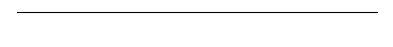# Ticks module

The `ticks` module adds a couple of functions to `matplotlib.axes.Axes` as shortcuts to the locators and formatters provided by `matplotlib.ticker`.

Here, their usage is demonstrated for the x-axis, but of course the equivalent functions for the y-axis exist as well. Simply replace `xticks` by `yticks` in the function name.

``````import numpy as np
import matplotlib.pyplot as plt
import plottools.ticks

fig, ax = plt.subplots()
``````

## Increments

``````ax.set_xticks_delta(0.5)
``````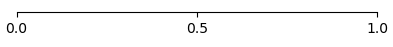## Custom format

``````ax.set_xticks_format('%04.1f')
``````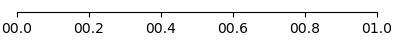## SI unit prefixes

``````ax.set_xscale('log')
ax.set_xlim(1e-6, 1e0)
ax.set_xticks_prefix()
``````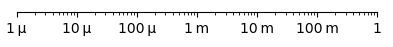## Fractions

``````ax.set_xlim(-1, 1)
ax.set_xticks_fracs(4)
``````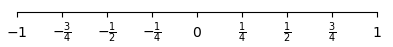## Multiples of Pi

``````ax.set_xlim(-np.pi, 2*np.pi)
ax.set_xticks_pifracs(2)
``````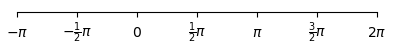## Pi in the nominator

``````ax.set_xlim(0, 4*np.pi/3)
ax.set_xticks_pifracs(3, True)
``````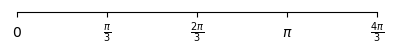## Fixed locations

``````ax.set_xticks_fixed((0, 0.3, 1))
``````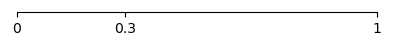## Fixed locations and labels

``````ax.set_xticks_fixed((0, 0.5, 1), ('a', 'b', 'c'))
``````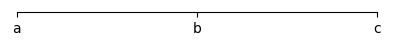## No tick labels

``````ax.set_xticks_blank()
``````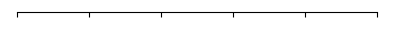You most likely want to use `common_xlabels()` from the `axes` module.

## No ticks at all

``````ax.set_xticks_off()
``````